Thermodynamics and Propulsion

17.2 Combined Conduction and Convection

We can now analyze problems in which both conduction and convection occur, starting with a wall cooled by flowing fluid on each side. As discussed, a description of the convective heat transfer can be given explicitly as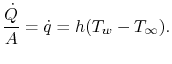This could represent a model of a turbine blade with internal cooling. Figure 17.6 shows the configuration.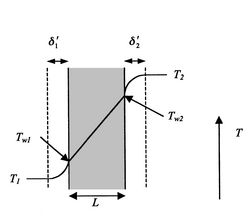The heat transfer in fluid 1 is given bywhich is the heat transfer per unit area to the fluid. The heat transfer in fluid 2 is similarly given byAcross the wall, we have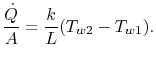The quantityis the same in all of these expressions. Putting them all together to write the known overall temperature drop yields a relation between heat transfer and overall temperature drop,:(17..20)

We can define a thermal resistance,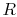, as before, such that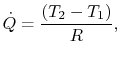whereis given by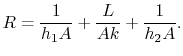(17..21)

Equation (17.21) is the thermal resistance for a solid wall with convection heat transfer on each side.

For a turbine blade in a gas turbine engine, cooling is a critical consideration. In terms of Figure 17.6,is the combustor exit (turbine inlet) temperature and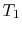is the temperature at the compressor exit. We wish to find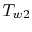because this is the highest metal temperature. From (17.20), the wall temperature can be written as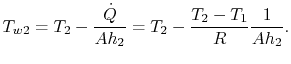(17..22)

Using the expression for the thermal resistance, the wall temperatures can be expressed in terms of heat transfer coefficients and wall properties as(17..23)

Equation (17.23) provides some basic design guidelines. The goal is to have a low value of. This means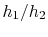should be large,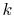should be large (but we may not have much flexibility in choice of material) and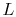should be small. One way to achieve the first of these is to havelow (for example, to flow cooling air out as in Figure 17.1 to shield the surface).

A second example of combined conduction and convection is given by a cylinder exposed to a flowing fluid. The geometry is shown in Figure 17.7.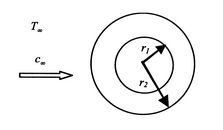For the cylinder the heat flux at the outer surface is given byThe boundary condition at the inner surface could be either a heat flux condition or a temperature specification; we use the latter to simplify the algebra. Thus,at. This is a model for the heat transfer in a pipe of radiussurrounded by insulation of thickness. The solution for a cylindrical region was given in Section 16.5.1 asUse of the boundary conditionyields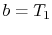.

At the interface between the cylinder and the fluid,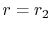, the temperature and the heat flow are continuous. (Question: Why is this? How would you argue the point?)(17..24)

Plugging the form of the temperature distribution in the cylinder into Equation (17.24) yields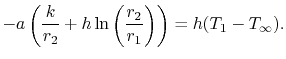The constant of integration,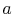, is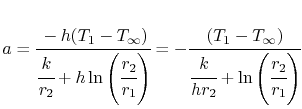and the expression for the temperature is, in normalized non-dimensional form,(17..25)

The heat flow per unit length,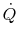, is given by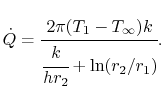(17..26)

The units in Equation (17.26) are W/m-s.

A problem of interest is choosing the thickness of insulation to minimize the heat loss for a fixed temperature difference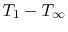between the inside of the pipe and the flowing fluid far away from the pipe. (is the driving temperature distribution for the pipe.) To understand the behavior of the heat transfer we examine the denominator in Equation (17.26) as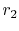varies. The thickness of insulation that gives maximum heat transfer is given by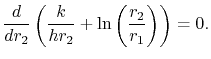(17..27)

(Question: How do we know this is a maximum?)

From Equation (17.27), the value offor maximumis thus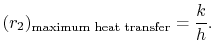(17..28)

Ifis less than this, we can add insulation and increase heat loss. To understand why this occurs, consider Figure 17.8, which shows a schematic of the thermal resistance and the heat transfer. Asincreases from a value less than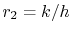, two effects take place. First, the thickness of the insulation increases, tending to drop the heat transfer because the temperature gradient decreases. Secondly, the area of the outside surface of the insulation increases, tending to increase the heat transfer. The second of these is (loosely) associated with theterm, the first with the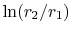term. There are thus two competing effects which combine to give a maximumat.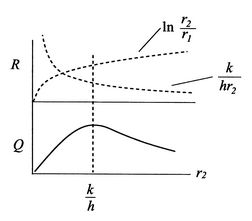Muddy Points

In the expression, what is? (MP 17.4)

It seems that we have simplified convection a lot. Is finding the heat transfer coefficient,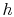, really difficult? (MP 17.5)

What does the K'' in the contact resistance formula stand for? (MP 17.6)

In the equation for the temperature in a cylinder (17.25), what is r?'' (MP 17.7)

UnifiedTP